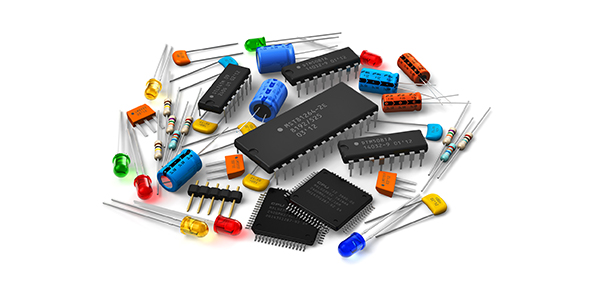# Interesting Quiz On Amplifiers

21 Questions | Total Attempts: 349SettingsAn amplifier, or amp, is an electronic device that can be used to increase the power of a signal. We typically use it in machines to enhance volume, i. E. Speakers. What do you know about them?

• 1.
In a push-pull amplifier, what makes an input signal two of equal amplitude and opposite polarity?
• A.

Ripple Converter

• B.

White Cathode Follower

• C.

Phase Splitter

• D.

Atom Splitter

• 2.
Which of these is not an actual twentieth-century amplifier brand?
• A.

Marshall

• B.

Real Time

• C.

Peavey

• D.

Fender

• 3.
For vacuum tubes used in power amplifiers, the filament power supply is typically how many volts?
• A.

115 Volts Ac

• B.

6.3 Volts Ac Or Dc

• C.

400 Volts Ac

• D.

50 Volts Dc

• 4.
Which one of the following is not a common amplifier distortion?
• A.

Slew Rate Limiting

• B.

Intermodulation Distortion

• C.

Apochromatic Distortion

• D.

Total Harmonic Distortion

• 5.
A vacuum tube predominately amplifies which of the following?
• A.

Voltage

• B.

Resistance

• C.

Current

• D.

Vacuum

• 6.
What famous amplifier was published in the magazine Wireless World in 1947, and set a standard of performance that was years ahead of its time?
• A.

The Marantz Model 9

• B.

• C.

The Williamson

• D.

Ampzilla

• 7.
A tetrad vacuum tube utilizes how many grids?
• A.

1

• B.

3

• C.

7

• D.

2

• 8.
How many grids does a pentode vacuum tube have?
• A.

3

• B.

5

• C.

2

• D.

1

• 9.
In Great Britain, what is the vacuum tube known as?
• A.

Valve

• B.

Audion

• C.

Glass Transistor

• D.

Faucet

• 10.
A Futterman amplifier is unique due to what fact?
• A.

It Does Not Use An Audio Output Transformer

• B.

Uses Only One Vacuum Tube

• C.

All Of These Choices

• D.

Uses Global Positive Feedback

• 11.
Which statement best describes Class A amplifier operation?
• A.

Current Flows During Both The Positive And Negative Half Cycles For A Conduction Angle Of 360 Degrees

• B.

Current Only Flows During The Positive Half Cycle Of The Input Waveform

• C.

Current Only Flows During The Negative Half Cycle Of The Input Waveform

• D.

Current Only Flows During 1/4 Of The Positive Half Cycle Of The Input Waveform

• 12.
What stage of a vacuum tube amplifier is the most sensitive to hum and noise?
• A.

Input Stage

• B.

Phase Splitter

• C.

Output Stage

• D.

Driver Stage

• 13.
What does the term "hybrid" mean in the context of power amplifier technology?
• A.

A Power Amp That Combines Vacuum Tubes And Solid State Devices In The Input And Output Stages

• B.

An Amplifier That Utilizes State Of The Art Technology

• C.

A Power Amp That Combines Ics And Discrete Devices

• D.

A Mix Of Bipolar, Jfet, And Or Mosfet Transistors

• 14.
What is the main difference between MOSFET output devices and bipolar junction transistors?
• A.

Bipolar Transistors Are Considerably Larger And More Expensive

• B.

Mosfets Are Biased By Voltage Rather Than Current

• C.

Mosfets Run Cooler, So Therefore More Efficiently

• D.

Bipolar Transistors Have Higher Crossover Distortion

• 15.
What does the term "bridging" mean in amplifier terminology?
• A.

None Of These Choices

• B.

Refers To Driving Two Pairs Of Loudspeakers With One Stereo Amplifier

• C.

A Way Of Connecting A Stereo Amplifier To Double Output Power

• D.

When Two Loudspeakers Are Connected In Series To One Channel Of A Power Amp

• 16.
Why are field effect transistors (FETs) commonly used in the input stage of a power amplifier?
• A.

They Offer High Input Impedance And Low Noise

• B.

They Make The Circuit More Linear And Lower Distortion

• C.

They Offer Better Conversion Efficiency Than Bipolar Junction Transistors

• D.

All Of These Choices

• 17.
A power amplifier with a high output impedance will have a low damping factor. What is the definition of damping factor?
• A.

The Ratio Between The Input And Output Voltages

• B.

The Ratio Of Output Current To Output Voltage

• C.

The Amount Of Negative Feedback In Db

• D.

The Ability To Control Woofer Cone Motion In A Loudspeaker

• 18.
Which of the following is not a true fact about class B amplifiers?
• A.

They Are Not Used As Audio Frequency Amplifiers Due To Crossover Distortion

• B.

They Have A Higher Efficiency Than Class C Amplifiers

• C.

They Have A Higher Efficiency Than Class A Amplifiers

• D.

They Have A Conduction Angle Of 180 Degrees

• 19.
Which of the following is a class A amplifier?
• A.

Mesa/Boogie Heartbreaker

• B.

Fender Twin

• C.

Marshall Jcm 2000

• D.

Vox Ac30

• 20.
What guitar and amplifier company makes the "Can" amp?
• A.

Ibanez

• B.

Epiphone

• C.

Peavey

• D.

Fender

• 21.
In a spread spectrum communication system, what is an approximation of processing gain?
• A.

Spread Spectrum Cannot Yield Processing Gain

• B.

If Bandwidth Divided By Data Rate

• C.

Chip Rate Divided By Data Rate

• D.

The Code Repeat-Length Times Beta Factor

Related TopicsBack to top